# 2059: Modified Bayes' Theorem

 Modified Bayes' Theorem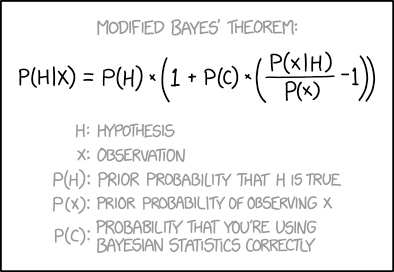Title text: Don't forget to add another term for "probability that the Modified Bayes' Theorem is correct."

## ExplanationThis explanation may be incomplete or incorrect: Created by a PROBABLE HYPOTHESIS. Please edit the explanation below and only mention here why it isn't complete. Do NOT delete this tag too soon.If you can address this issue, please edit the page! Thanks.
Bayes' Theorem is an equation in statistics that gives the probability of a given hypothesis accounting not only for a single experiment or observation, but also for your existing knowledge about the hypothesis, i.e. it's prior probability. Randall's modified form of the equation also purports to account for the probability that you are indeed applying Bayes' Theorem itself correctly by including that as a term in the equation.

Bayes' theorem is stated as:$P(A\mid B) = \frac{P(B \mid A) \, P(A)}{P(B)}$,

where

•$P(A\mid B)$ is the likelihood of event$A$ occurring given that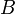$B$ is true
•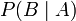$P(B\mid A)$ is the likelihood of event$B$ occurring given that$A$ is true
•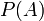$P(A)$ and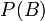$P(B)$ are the probabilities of observing$A$ and$B$ independently

If P(C)=1 the modified theorem reverts to the original Bayes' theorem (which makes sense, as a probability one would mean that you are using Bayes' theorem correctly). If P(C)=0 the modified theorem becomes P(H|X) = P(H), which says that the probability of your hypothesis is not affected by the result of the observation.

## TranscriptThis transcript is incomplete. Please help editing it! Thanks.
Modified Bayes' theorem:

P(H|X) = P(H) * (1 + P(C) * (P(X|H)/P(X) - 1))

H: Hypothesis
X: Observation
P(H): Prior probability that H is true
P(X): Prior propability of observing X
P(C): Propability that you're using Bayesian statistics correctlyadd a comment! ⋅add a topic (use sparingly)! ⋅refresh comments!

# Discussion

Right now the layout is awful:

"If$P(C)=1$ the..."
should look like this:
"If P(C)=1 the..."

But there is more wrong right now. Look at a typical Wikipedia article, the Math-extension should be used for formulas but not in the floating text. --Dgbrt (talk) 20:03, 15 October 2018 (UTC)

Credit for a good explanation though. It made perfect sense to me, even though I didn't understand it. 162.158.167.42 04:14, 16 October 2018 (UTC)
I fixed the layout and tried to enhance the explanation. --Dgbrt (talk) 20:19, 17 October 2018 (UTC)

I removed this, because it makes no sense:

As an equation, the rewritten form makes no sense.$P(H \mid X) = P(H)(1-P(C)) + P(H \mid X)P(C)$ is strangely self-referential and reduces to the piecewise equation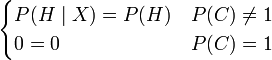$\begin{cases}P(H \mid X) = P(H) & P(C) \neq 1 \\ 0 = 0 & P(C) = 1 \end{cases}$. However, the Modified Bayes Theorem includes an extra variable not listed in the conditioning, so a person with an AI background might understand that Randal was trying to write an expression for updating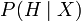$P(H \mid X)$ with knowledge of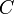$C$ i.e.$P(H \mid X,C)$, the belief in the hypothesis given the observation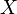$X$ and the confidence that you were applying Bayes' theorem correctly$C$, for which the expression$P(H \mid X,C) = P(H)(1-P(C)) + P(H \mid X)P(C)$ makes some intuitive sense.

Between removing it and posting here, I think that I've figured out what it's saying. But it comes down to criticizing a mistake made in an earlier edit by the same editor, so I'll just fix that mistake instead.

TobyBartels (talk) 13:03, 16 October 2018 (UTC)

What about examples of correct and incorrect use of Bayes' Theorem? I don't feel equal to executing that, but DNA evidence in a criminal case could be illuminating. As a sketch, it may show that of 7 billion people alive today, the blood at the scene came from any one of just 10,000 people of which the accused is one. Interesting, but not absolute. At least 9,999 of the 10,000 are innocent. Probability of mistake or malfeasance by the testing laboratory also needs to be considered. Then there's sports drug testing, disease screening with imperfect tests and rare true positives, etc. [email protected] 141.101.76.82 09:42, 17 October 2018 (UTC)

Where is the next comic button for this page? IYN (talk) 15:46, 17 October 2018 (UTC)

Please be patient for that button, it always appears a little bit late since a few days ago when a new comic is out. Right now you always can click the Latest comic link at the menu. I'm aware of this minor issue. --Dgbrt (talk) 20:16, 17 October 2018 (UTC)